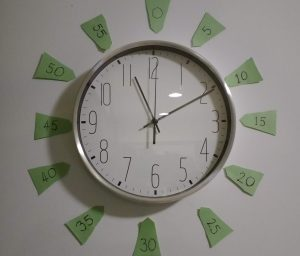# Skip Counting Fives

Learning to match the fives with the numbers 1 to 12 is key to telling the time on an analogue cock. For our kid, this was the first introduction to a multiplication table – a mathematical tool useful in the context. But first, we spent a long time with the number pairs. In a set of five lessons on telling the time, we used ‘the fives’ in all of them, but only discussed multiplication; thesymbol and thesymbol in lesson #4.

# A set of Cards

To print: Multiples 5

In this task, print the numbers 1 – 12 on a different colour sheet from the multiples of 5.

1. Shuffle the cards together then scatter them face up on the floor. Make pairs, eg, it takes ‘3’ hands to count ’15’ fingers. etc.

2. Memory pairs. Nice to have the ‘answers’ visible somewhere in the room. We had this applet on a tablet with ‘minutes’ showing beside us.

3. Go fish (for pairs). Start with four cards each, up to three players. Again, nice to have the ‘answers’ to hand.

# Rectangles

To print: Rectangles Rows of 5

1. We did a scavenger hunt. We gave each rectangle a name, made up a story about spying rectangle-aliens, wrote a list with ‘name & number of boxes’. When each rectangle was found it was identified by the number of boxes then its name was scored off the list.

2. Making rectangles: